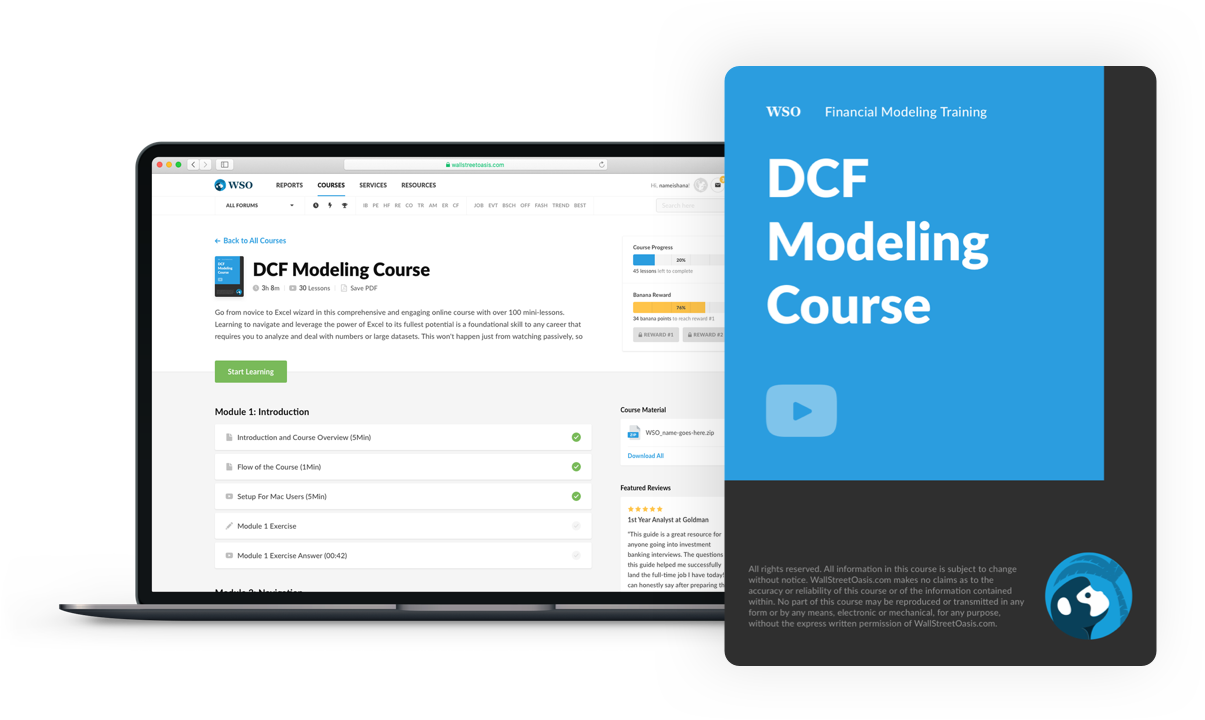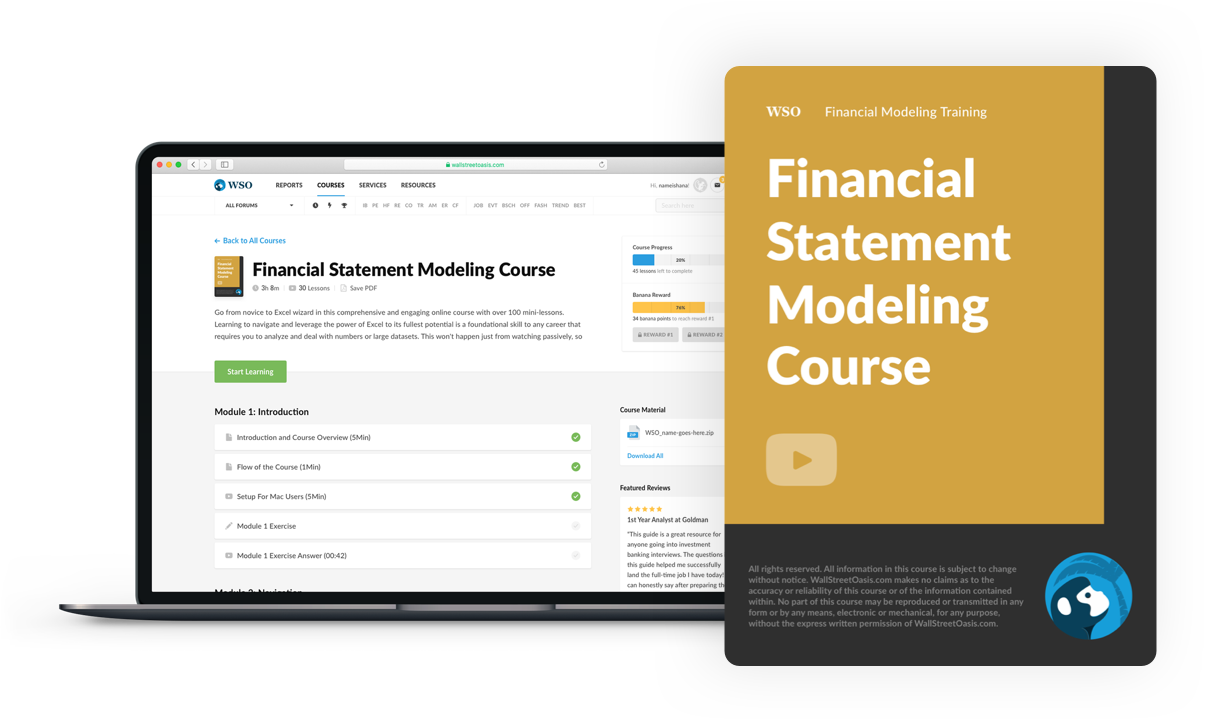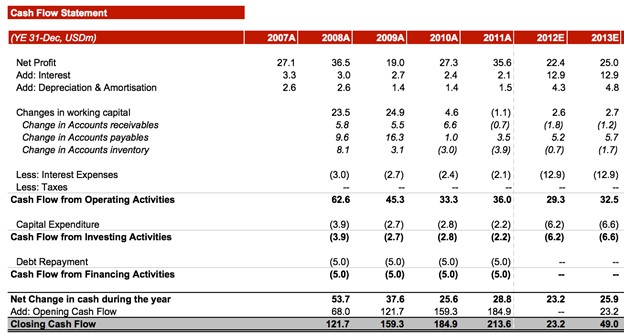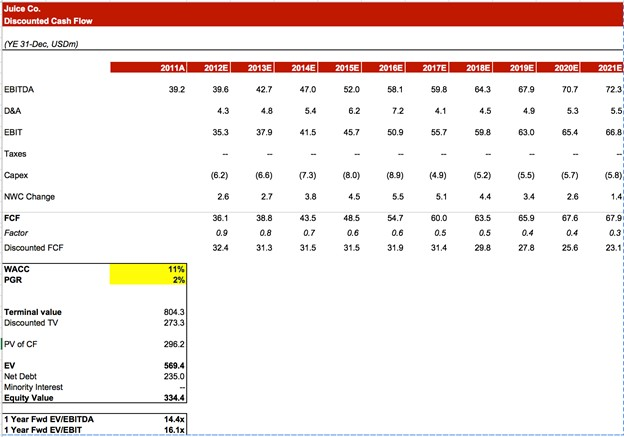# DCF Model Training Free Guide

A free guide on how to build DCF models in Excel

The discounted cash flow (DCF) model is one of the most important and widely used financial modeling methods to value a company. It requires estimating the total value of all future cash flows (both inflows and outflows) and then discounting them (usually using Weighted Average Cost of Capital – WACC) to find the net present value (NPV). The DCF method of valuation is based on the belief that the value of a business equals the total discounted cash flows it generates.This model uses estimates to calculate the future values and, as a result, could be unreliable if the estimates are inaccurate (garbage in = garbage out) which is it is recommended to use various valuation methods to value an asset. Some of these other methods include trading multiples, which would fall under the category of comparable analysis, as well as leveraged buyout (LBO) analysis.

## DCF vs. Cash flow vs. Free cash flow

Cash flow (CF) is the net amount of cash and cash equivalents (like short-term government bonds and treasury bills) being transferred in and out of an organization, where inflow indicates cash being received while outflow means money being spent.

Free cash flow (FCF) is cash flow that is generated from operations, free from any encumbrances. It is primarily used to determine the present value of a company, meaning how much money is available to investors through the business's operations after accounting for expenses like tax, operating expenses (OPEX), and capital expenditures (CAPEX). Thus FCF is more specific than regular cash flow.

FCF can be categorized as levered and unlevered, a topic discussed in this forum post. Discounted cash flow models generally tend to use unlevered FCF (UFCF), which considers the cash flows available to be debt and equity holders and is used to calculate the enterprise value of a company (value of a company as a whole, including debt and equity).

On the other hand, levered FCF is the cash available to only equity holders and hence is used to calculate the equity value (debt is ignored).

To understand the difference between levered and unlevered free cash flow, please watch our video from our DCF Modelling Course, which explores this topic.

## WSO’s TLDR

In the simplest terms, discounted cash flow (DCF) is the sum of all future cash flows discounted to arrive at the value of asset generating them.

We discount future cash flow because the value of cash appreciates over time, which means that money received today is worth more than money received in the future.

For example, having \$20 today would be worth more than having \$20 in the future. This is because you can deposit the \$20 you have now at a bank to earn interest which increases its value.### Everything You Need To Master DCF Modeling

To Help you Thrive in the Most Prestigious Jobs on Wall Street.

## Discounted cash flow calculation: Formula

The DCF formula is given as follows:

DCF = CF1/(1+r)1 + CF2/(1+r)2 + . . . + CFn(1+r)n

where,
DCF
= Discounted cash flow
CFi = Cash flow in the period i, so the first cash flow in the first period is CF1
r
= Interest rate or discount rate per annum
n = Time in years of the final cash flowIt can be used to value multiple asset types such as:

• Projects or investment opportunities
• Shares
• Bonds
• Property

Performing calculations of any kind can be tedious, and this is especially true with the DCF formula. Fortunately, analysts can use Excel to do the heavy lifting for most calculations. If you would like to learn some tips and tricks to improve your excel skills, please look at our Excel Modeling Course which covers various topics to make you an Excel whiz like this one which covers conditional formatting:### Everything You Need To Master DCF Modeling

To Help you Thrive in the Most Prestigious Jobs on Wall Street.

## Discounted cash flow model: How to make one?

Like other models, such as the financial and accretion dilution models, there are multiple steps to create a DCF model.

Here is a demo video from our financial statement modeling course illustrating the linking of cash flows to the three statements which is one of the final steps in the process.### Everything You Need To Master Financial Statement Modeling

To Help you Thrive in the Most Prestigious Jobs on Wall Street.

### Projecting future Unlevered Free Cash Flows (UFCF)

As the name suggests, a discounted cash flow model requires projections of future cash flows to discount them to a present value. Hence, we must first project them using the cash flow statement (CFS). If the DCF model is built after a financial model, the projected CFS should already have been created which can be used directly in our model.

However, let's suppose that the model is being created from scratch in which case, we must project it first from the balance sheet (BS) and income statement (IS), as well as the supporting schedules.

Once we have the cash flow statement, we can calculate free cash flow by using the formula:

FCF = CFO - CapEx

where,
CFO = Cash flow from operations
CapEx = Capital expenditure### Determining the discount rate

The discount rate (or required rate of return) can be taken as an assumed percentage or be calculated using the weighted average cost of capital (WACC). The WACC is a financial metric used to measure the cost of capital to a firm.

The discount rate represents the riskiness of the free cash flow generated. A riskier project should be discounted using a higher discount rate and vice versa. It is also affected by the firm's capital structure, as the use of leverage increases the required rate of return demanded by the equity shareholders.### Discounting cash flows

Now we need to calculate the discounted cash flows generated by the investment, which is done by plugging the cash flow for each period into the DCF formula: Sum of cash flows of all periods / (1 + discount rate).

### Calculating terminal value

The terminal value (TV) is the value of a business, project, or asset beyond the forecasted period. TV is used because we cannot accurately estimate the cash flow generated by an investment to perpetuity (indefinitely). In our model, the TV would be calculated at the end of the period for which the DCF model is being prepared.

There are two ways to calculate terminal value:

• Gordon Growth Model, otherwise known as the perpetual growth model, and
• The exit multiple/terminal multiple methods

The Gordon Growth method assumes that cash flow will continue to grow at a constant rate after the forecast period.

For example, suppose a business has a cash flow of \$10 million expected to grow at 1% into eternity and a WACC of 11%. In that case, their terminal value will be calculated as \$100m (\$10 million / (11% - 1%)).The exit multiple approach uses a different assumption in valuing the business. Rather than expecting a constant growth rate, this method simply uses a trading multiple, typically the EV / EBITDA ratio, to estimate what the company will be worth based on the ratio at which other comparable companies in the same industry are trading

For instance, if the organization has \$5 million of EBITDA and the other companies are trading at 5x, you multiply these two values to get \$25 million. Regardless of which method is used, the terminal value would be discounted to the present day to get its net present value.

Therefore, the terminal value can usually make up a large amount of an organization's NPV in the DCF model, especially for relatively lower time frames like five years.

Here is a short video on methods for calculating a terminal value from our DCF Modelling Course to help you grasp the concepts better.### Everything You Need To Master DCF Modeling

To Help you Thrive in the Most Prestigious Jobs on Wall Street.

### Determine the value and perform sensitivity analysis

To complete the discounted cash flow model, we add the present value of our cash flows with the terminal value that we calculated to arrive at present value of the asset.

As a final touch to the model, analysts can perform sensitivity analysis to ensure the model's reliability, similar to how financial models are tested. Essentially, changing the underlying assumptions (like those used in the financial statements) will provide a range of values that the business could be worth. For instance, the discount range or the projected cash flows could be changed depending on the assumptions made.

## WSO’s free DCF template

WSO provides its readers with a free DCF model template that you can use to build your model with different assumptions and practice the steps that have been explained.

Here is a short video on the DCF model that you can watch to consolidate your understanding of creating one:

For a more in-depth explanation of the process, please watch this video that provides an in-depth half-hour explanation on how to perform DCF calculation:### Everything You Need To Master DCF Modeling

To Help you Thrive in the Most Prestigious Jobs on Wall Street.

## Example of DCF Modeling

To aid your understanding of how to create and interpret a DCF model, we will go through the WSO discounted cash flow template below.

1. We have ten years of projected free cash flows (FCF), which we discount with a WACC of 11% to find the discounted FCF of each year.
2. After this, the terminal value is calculated using the perpetual growth rate (PGR) of 2%. Similar to the FCF, this is discounted using the same WACC to find the discounted TV.
3. All the discounted FCF are totaled to find the present value of cash flows (PV of CF).
4. The discounted TV and PV of CF are added together to find the enterprise value (EV).
5. As an additional step, we can find the equity value by subtracting net debt and minority interest from the enterprise value.## Issues with the DCF model

While the discounted cash flow model is very useful as it can quantify a sophisticated deal by providing investors with a number representing the worth of a company or project, it is not without disadvantages.

### Inaccurate cash flow and CapEx projections

As with most valuation methods, assumptions are used to make projections which are subject to inaccuracies. However, with the DCF model, the usual time frame of five to ten years means that even slight errors in the assumptions for the first or second year could compound and lead to a drastically different result. Each period is linked to the previous one, so an error in any period would render all forecasts of the future periods useless.

This linked nature of DCF models also introduces difficulties as analysts making assumptions for the later years have less accurate information to base off, increasing the degree of uncertainty. Since DCF models are intended to provide long-term valuations instead of short-term ones, this is a prominent drawback of the model.

Part of projecting free cash flows is estimating a company's capital expenditures for each period. In a similar vein to making cash flow projections, analysts who estimate CapEx will face the difficulties of not having sufficient accurate information, making CapEx projections susceptible to a high degree of inaccuracy, especially for the later years.### Fixed discount rate and growth rate

Another problem with this model is that it assumes both the discount rate and growth rate of an organization are fixed, which is hardly ever the case in reality. Constant fluxes in the economy and developments within an organization would lead to increases and decreases in both these rates.

Focusing on the discount rate also presents the issue of how an analyst chooses to calculate it. While using the WACC is a common method, there are other ways to determine the discount rate, creating the complication of different analysts and firms using different ways of finding the discount rate. Moreover, regardless of what method is used, all approaches tend to be more theoretical than practical for real-world applications.

Another assumption that is unrealistic is the assumption of constant growth rate into perpetuity while calculating the terminal value. One method to address this is to use the economy's long-term growth rate, which is around 4 percent for the United States. However, this is not foolproof because growth is anything but constant over a long period.

### Solution to issues of the DCF model

As with all forms of financial and valuation modeling, it is best to combine multiple methods and data points to better understand the concept behind the model. These include using:

In addition to this, since DCF models can have a large variation in results depending on the inputs used, analysts can provide a range of valuations that may be more accurate than pinpointing a number.### Everything You Need To Master DCF Modeling

To Help you Thrive in the Most Prestigious Jobs on Wall Street.